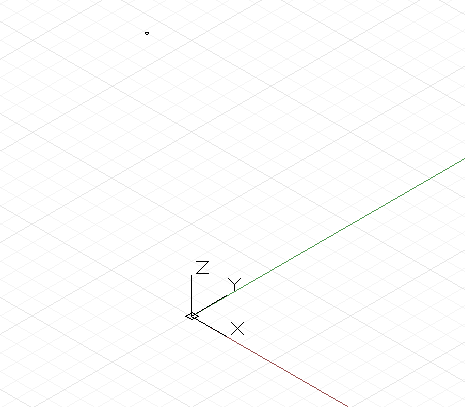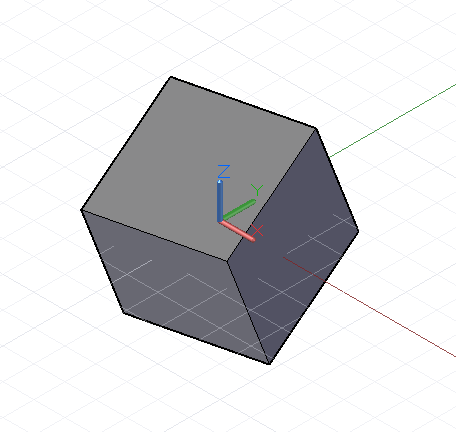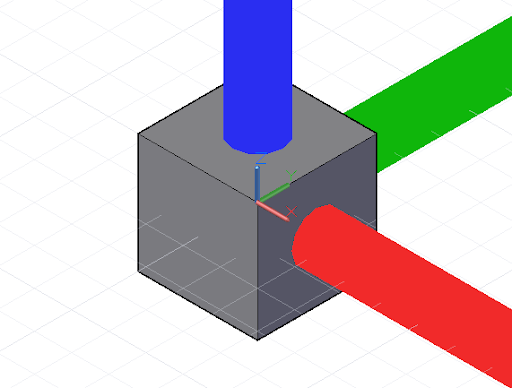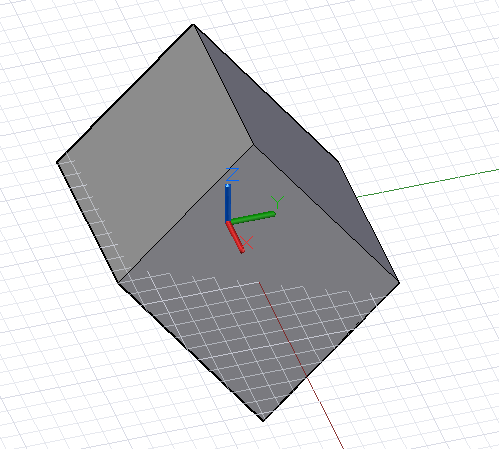# Translation, Rotation, and Other Transformations

Certain geometry objects can be created by explicitly stating x, y, and z coordinates in three-dimensional space. More often, however, geometry is moved into its final position using geometric transformations on the object itself or on its underlying CoordinateSystem.

The simplest geometric transformation is a translation, which moves an object a specified number of units in the x, y, and z directions.``````// create a point at x = 1, y = 2, z = 3
p = Point.ByCoordinates(1, 2, 3);

// translate the point 10 units in the x direction,
// -20 in y, and 50 in z
// p2’s new position is x = 11, y = -18, z = 53
p2 = p.Translate(10, -20, 50);
``````

While all objects in Dynamo can be translated by appending the .Translate method to the end of the object’s name, more complex transformations require transforming the object from one underlying CoordinateSystem to a new CoordinateSystem. For instance, to rotate an object 45 degrees around the x axis, we would transform the object from its existing CoordinateSystem with no rotation, to a CoordinateSystem which had been rotated 45 degrees around the x axis with the .Transform method:``````cube = Cuboid.ByLengths(CoordinateSystem.Identity(),
10, 10, 10);

new_cs = CoordinateSystem.Identity();
new_cs2 = new_cs.Rotate(Point.ByCoordinates(0, 0),
Vector.ByCoordinates(1,0,0.5), 25);

// get the existing coordinate system of the cube
old_cs = CoordinateSystem.Identity();

cube2 = cube.Transform(old_cs, new_cs2);
``````

In addition to being translated and rotated, CoordinateSystems can also be created scaled or sheared. A CoordinateSystem can be scaled with the .Scale method:``````cube = Cuboid.ByLengths(CoordinateSystem.Identity(),
10, 10, 10);

new_cs = CoordinateSystem.Identity();
new_cs2 = new_cs.Scale(20);

old_cs = CoordinateSystem.Identity();

cube2 = cube.Transform(old_cs, new_cs2);
``````

Sheared CoordinateSystems are created by inputting non-orthogonal vectors into the CoordinateSystem constructor.``````new_cs = CoordinateSystem.ByOriginVectors(
Point.ByCoordinates(0, 0, 0),
Vector.ByCoordinates(-1, -1, 1),
Vector.ByCoordinates(-0.4, 0, 0));

old_cs = CoordinateSystem.Identity();

cube = Cuboid.ByLengths(CoordinateSystem.Identity(),
5, 5, 5);

new_curves = cube.Transform(old_cs, new_cs);
``````

Scaling and shearing are comparatively more complex geometric transformations than rotation and translation, so not every Dynamo object can undergo these transformations. The following table outlines which Dynamo objects can have non-uniformly scaled CoordinateSystems, and sheared CoordinateSystems.

Class Non-Uniformly Scaled CoordinateSystem Sheared CoordinateSystem
Arc No No
NurbsCurve Yes Yes
NurbsSurface No No
Circle No No
Line Yes Yes
Plane No No
Point Yes Yes
Polygon No No
Solid No No
Surface No No
Text No No